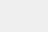# Python下的Numpy函数对应关系

小编写这篇文章的主要目的，是为了帮助在Python中学习的人们，帮助他们能够更好更快速的进行学习。今天，我就给大家介绍一下，关于Python下Numpy函数对应关系的实例，大家要仔细阅读哦。

Matlab函数对应关系(Numpy)

首先给出官网链接，其中详细说明了在Python下如何用Numpy实现Matlab下相同的函数功能。

博主在用Python撰写代码的时候，想用Python实现在Matlab下某个函数的功能（比如Repmat函数），但是当使用语句

```　from numpy.matlib import repmat
A=repmat(B,1,2)```

调用工具包时，虽然可以正常实现功能，但是PyCharm下报出警告：

Importing from numpy.matlib is deprecated since 1.19.0.The matrix subclass is not the recommended way to represent matrices or deal with linear algebra(see https://docs.scipy.org/doc/numpy/user/numpy-for-matlab-users.html).Please adjust your code to use regular ndarray.

随后，通过查阅资料，发现是因为从1.19.0版本后，numpy就不再推荐使用numpy.matlib来实现对应功能了。

因此，大家可以对照官网上的说明来实现替换。

这里截取一小部分：其他的可以从官网上面找到。

Numpy与Matlab互转操作

在日常使用中，matlab作为我们机器学习以及深度学习的模型训练使用的工具，而线上使用python实现模型落地。因为不可避免常遇到matlab与numpy之间有些操作需要相互转换。

1、常用操作（参考numpy官方说明）2、线性变换（参考numpy官方说明）以上就是小编的个人经验，希望能够给各位读者带来帮助。